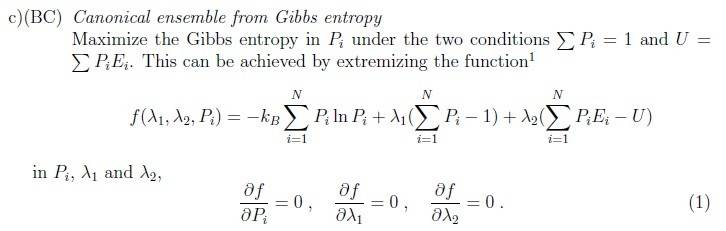# Maximizing Gibbs Entropy in Canonical Ensemble

Hey,

Here is the problem:The method by which we solve is by Langrange Multipliers, and so I believe I found the derivative of f with respects to P(i) but I have two quantities I'm sure what they equal:

Summations over i=1 to N : Ʃln(P(i)) and ƩE(i)

Thanks for any help,
S